ayoob

•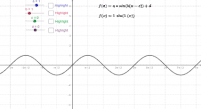Transforming a sin (b*x) + c

Activity

ayoob

•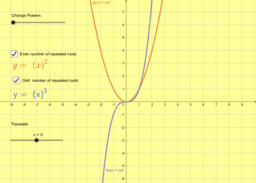Repeated Roots

Activity

ayoob

•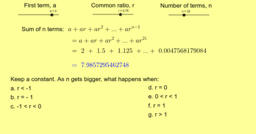Infinite Geometric Series

Activity

ayoob

•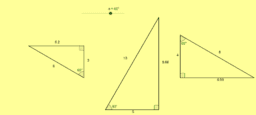3 Right-Angled Triangles

Activity

ayoob

•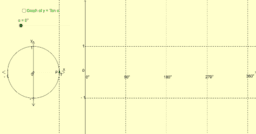Unit circle and Tangent function

Activity

ayoob

•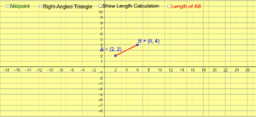Midpoint and Length of a Line Segment

Activity

ayoob

•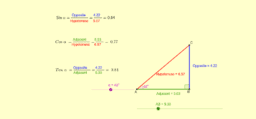Trigonometric Ratios

Activity

ayoob

•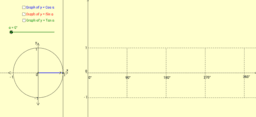Unit Circle and the Trigonometric Functions

Activity

ayoob

•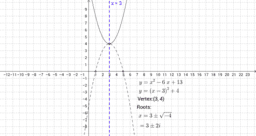Complex Numbers

Book

ayoob

•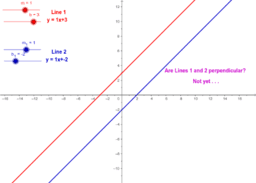Coordinate Geometry

Book

ayoob

•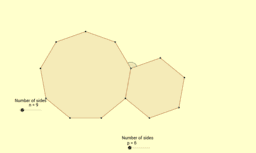Angles and Polygons

Book

ayoob

•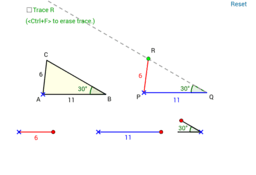Trigonometry

Book

ayoob

•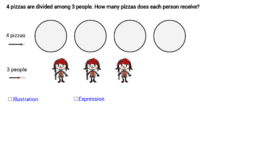Fractions

Book

ayoob

•Combined Polygons

Activity

ayoob

•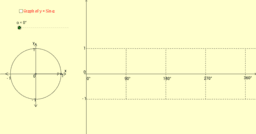Unit Circle and the Sine function

Activity

ayoob

•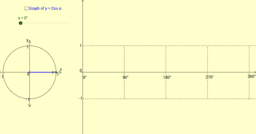Unit circle and cosine function

Activity

ayoob

•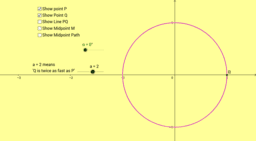Two Points on a Circle_Parametric Equations

Activity

ayoob

•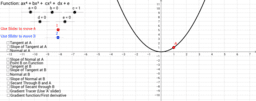Visualising Differentiation

Activity

ayoob

•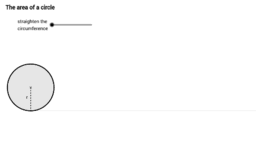Mensuration

Book

ayoob

•Calculus

Book

ayoob

•Pythagoras

Book

ayoob

•Book

ayoob